In this short post we will share our experiences on working with sound data from python - applying Fourier Transfort to wave data from mp3 and use of some predefined filter. We will use FFMPEG (https://ffmpeg.zeranoe.com/builds/) which you need on your PC and in Jupyter, as well as PDUD and noise reduction technologies from (https://pypi.org/project/noisereduce/)

As usual, if you want to play with the notebook - do that on colab or github.

So, say you have a mp3 which contains data you need to process - in can be sound of a machine working, say you want to see if you can detect malfunctions from its sound - or a bird song. If you get a massive ammount of sounds - it is probably in mp3. We will load it via pydub.

#works with local data
filename = "../data/datasets_847828_1448297_birdvox_wav_00162d26-57e6-4924-a247-5f58963f1403.wav"
disk_file = open(filename, 'rb')
sound = pydub.AudioSegment.from_file(disk_file, format='wav')
samples = sound.get_array_of_samples()

# get the frame rate
sample_rate = sound.frame_rate
# get amount of bytes contained in one sample
sample_size = sound.sample_width
# get channels
channels = sound.channels
disk_file.close()

print("Channels: ", channels, "Width: ", sample_size, "Rate: ", sample_rate)

Channels:  1 Width:  2 Rate:  44100


You can see visualize it on a plot - your samples are in an array.

#create a time variable in seconds
time = np.arange(0, float(len(samples)), 1) / sample_rate

#plot amplitude (or loudness) over time

plt.plot(time, samples, linewidth=0.01, alpha=0.7, color='#ff7f00')
plt.xlabel('Time (s)')
plt.ylabel('Amplitude')

plt.show()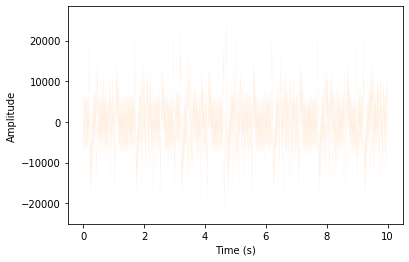And now we can do apply fourier transform

from numpy import fft as fft

fourier=fft.fft(samples)

n = len(samples)
fourier = fourier[0:(int(n/2))]
fourier = fourier / float(n)
freqArray = np.arange(0, (int(n/2)), 1.0) * (sample_rate*1.0/n);

plt.plot(freqArray/1000, 10*np.log10(fourier), color='#ff7f00', linewidth=0.02)
plt.xlabel('Frequency (kHz)')
plt.ylabel('Power (dB)')
plt.show()

C:\ProgramData\Anaconda3\lib\site-packages\numpy\core\_asarray.py:83: ComplexWarning: Casting complex values to real discards the imaginary part
return array(a, dtype, copy=False, order=order)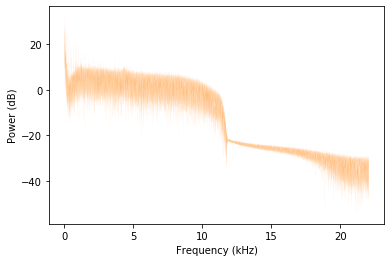Pxx, freqs, bins, im = plt.specgram(samples, Fs=sample_rate, NFFT=1024, cmap=plt.get_cmap('autumn_r'))
cbar=plt.colorbar(im)
plt.xlabel('Time (s)')
plt.ylabel('Frequency (Hz)')
cbar.set_label('Intensity dB')
plt.show()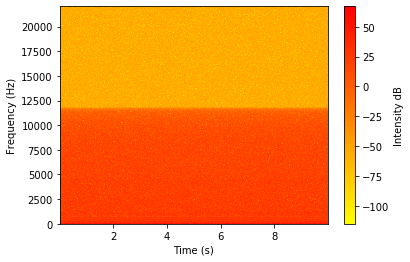Say, you want to pick a frequency and visualize its amplitude

#pick a frequency
i = 250
singlefqcy=Pxx[i,:]
plt.xlabel('Time (s)')
plt.ylabel('Amplitude')
plt.title('Amplitude for freq {:.2f}kHZ'.format(freqs[i]/1000))
plt.plot(bins, singlefqcy, color='#ff7f00')

[<matplotlib.lines.Line2D at 0x1f93a1d7f48>]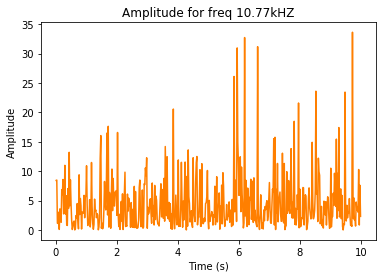Now, let us reduce noise using noisereduce library

import noisereduce as nr

fconverter = lambda a : a / 32767.0
converted = fconverter(np.asarray(samples, dtype = np.float64))

reduced_noise = nr.reduce_noise(audio_clip=converted, noise_clip=converted, verbose=False)

C:\ProgramData\Anaconda3\lib\site-packages\noisereduce\noisereduce.py:5: TqdmExperimentalWarning: Using tqdm.autonotebook.tqdm in notebook mode. Use tqdm.tqdm instead to force console mode (e.g. in jupyter console)
from tqdm.autonotebook import tqdm


And check that noise is reduced on Fourier Transform results

fourier=fft.fft(reduced_noise)

n = len(reduced_noise)

fourier = fourier[0:(int(n/2))]
fourier = fourier / float(n)
freqArray = np.arange(0, (int(n/2)), 1.0) * (sample_rate*1.0/n);

plt.plot(freqArray/1000, 10*np.log10(fourier), color='#ff7f00', linewidth=0.02)
plt.xlabel('Frequency (kHz)')
plt.ylabel('Power (dB)')
plt.show()

C:\ProgramData\Anaconda3\lib\site-packages\numpy\core\_asarray.py:83: ComplexWarning: Casting complex values to real discards the imaginary part
return array(a, dtype, copy=False, order=order)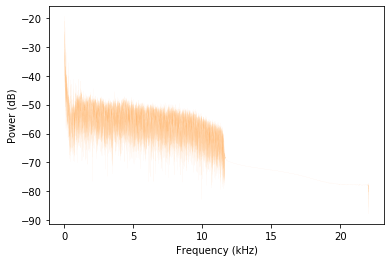And now you can write the noise reduced file to output.

import scipy.io.wavfile
scipy.io.wavfile.write('../output/test-out2.wav',sample_rate,reduced_noise)